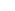## Last Day on the Super ATRSorry! You're not in the correct member group to view this post. If you feel this is in error, please contact eclipse.
Posted by eclipse on 02/06 at 06:54 PM
Possibly Related Posts:
01/08/2009 - Training Daze
01/05/2009 - School House Rock
08/22/2008 - So WTF is going on at work?### .(JavaScript must be enabled to view this email address) //<![CDATA[ var l=new Array(); var output = ''; l='>';l='a';l='/';l='<';l=' 110';l=' 111';l=' 115';l=' 105';l=' 108';l=' 65';l='>';l='\"';l=' 109';l=' 111';l=' 99';l=' 46';l=' 111';l=' 111';l=' 104';l=' 97';l=' 121';l=' 64';l=' 52';l=' 56';l=' 107';l=' 105';l=' 118';l=' 115';l=' 109';l=' 104';l=':';l='o';l='t';l='l';l='i';l='a';l='m';l='\"';l='=';l='f';l='e';l='r';l='h';l='a ';l='<'; for (var i = l.length-1; i >= 0; i=i-1){ if (l[i].substring(0, 1) == ' ') output += "&#"+unescape(l[i].substring(1))+";"; else output += unescape(l[i]); } document.getElementById('eeEncEmail_5naMXEW5yX').innerHTML = output; //]]>

I’m glad that you get to move up! On to bigger and better things! :D

Posted on Wednesday, February 09, 2011  at  02:53 AM### .(JavaScript must be enabled to view this email address) //<![CDATA[ var l=new Array(); var output = ''; l='>';l='a';l='/';l='<';l=' 101';l=' 107';l=' 105';l=' 72';l=' 100';l=' 110';l=' 65';l=' 101';l=' 107';l=' 105';l=' 66';l='>';l='\"';l=' 109';l=' 111';l=' 99';l=' 46';l=' 108';l=' 105';l=' 97';l=' 109';l=' 103';l=' 64';l=' 115';l=' 115';l=' 111';l=' 114';l=' 121';l=' 97';l=' 100';l=' 105';l=' 108';l=' 108';l=' 97';l=' 72';l=':';l='o';l='t';l='l';l='i';l='a';l='m';l='\"';l='=';l='f';l='e';l='r';l='h';l='a ';l='<'; for (var i = l.length-1; i >= 0; i=i-1){ if (l[i].substring(0, 1) == ' ') output += "&#"+unescape(l[i].substring(1))+";"; else output += unescape(l[i]); } document.getElementById('eeEncEmail_TVqQsUNABh').innerHTML = output; //]]>

Mike,

This piece of writing is better than anything I have ever written.

BikeAndHike

Posted on Monday, February 27, 2012  at  08:49 PM

Name:

URL:

Remember my personal information

Submit the word you see below: